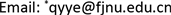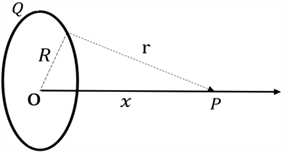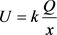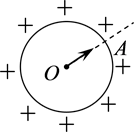﻿ 利用高考题做好高中与大学物理衔接 Link High School Physics with College Physics by Questions of College Entrance Examination

Vol. 07  No. 10 ( 2018 ), Article ID: 27337 , 6 pages
10.12677/ASS.2018.710256

Link High School Physics with College Physics by Questions of College Entrance Examination

Yeqing Liu, Shengkai Huang, Yajing Ye, Qingying Ye*

College of Physics and Energy, Fujian Provincial Key Laboratory of Quantum Manipulation and New Energy Materials, Fujian Normal University, Fuzhou FujianReceived: Oct. 10th, 2018; accepted: Oct. 23rd, 2018; published: Oct. 30th, 2018ABSTRACT

Currently, college entrance examinations are not only limited to the knowledge learned in the textbook, but also require students to master some ideas of college physics. In order to make students cope better with the college entrance examination, and adapt to the study of college physics quickly, the teacher can analyze the complex questions of the college entrance examinations, contrast the solution of high school to university, reform the educational model, and then make good connection between high school and university.

Keywords:High School Physics, College Physics, Questions of College Entrance Examination, Connection1. 引言

2. 高中与大学物理衔接存在的问题及后果

2.1. 存在的问题

2.2. 导致的后果

2.2.1. 解答高考题变得困难

2.2.2. 大学的学习将不顺利

3. 高中物理与大学物理的衔接策略

1) 常规解法：Figure 1. Title example

2) 微元法：

$\Delta U=k\frac{\Delta Q}{r}$

$U=\sum \Delta U=k\frac{\sum \Delta Q}{r}=k\frac{Q}{r}=k\frac{Q}{\sqrt{{R}^{2}+{x}^{2}}}$

3) 微积分法：

$\text{d}U=k\frac{\text{d}Q}{r}$

$U=k\frac{{\int }_{Q}\text{d}Q}{r}=k\frac{Q}{r}=k\frac{Q}{\sqrt{{R}^{2}+{x}^{2}}}$1) 常规解法：Figure 2. Title example

2) 大学解法：

${U}_{\text{0}}=k\frac{Q}{R}$ (1)

${U}_{M}={\int }_{r}^{\infty }E\cdot \text{d}r={\int }_{r}^{\infty }k\frac{Q}{{r}^{2}}\text{d}r=k\frac{Q}{r}$ (2)

${E}_{k0}+{U}_{0}q={E}_{k}+{U}_{M}q$ (3)

${E}_{k}={E}_{k0}+\frac{kQq}{R}-\frac{kQq}{r}$ (4)

4. 总结

Link High School Physics with College Physics by Questions of College Entrance Examination[J]. 社会科学前沿, 2018, 07(10): 1718-1723. https://doi.org/10.12677/ASS.2018.710256

1. 1. 陈奋策. 小议“大学物理题目改编为高考题” [J]. 福建教育学院学报, 2014, 15(2): 94-96.

2. 2. 刘爽. 从大学物理高度看高中物理教学[D]: [硕士学位论文]. 长春: 东北师范大学, 2010.

3. 3. 兰智高. “大学物理教学衔接问题”的研究述略[J]. 枣庄师范专科学校学报, 2002(2): 31-33.

4. 4. 梁灿彬. 电磁学[M]. 第三版. 北京: 高等教育出版社, 2013.